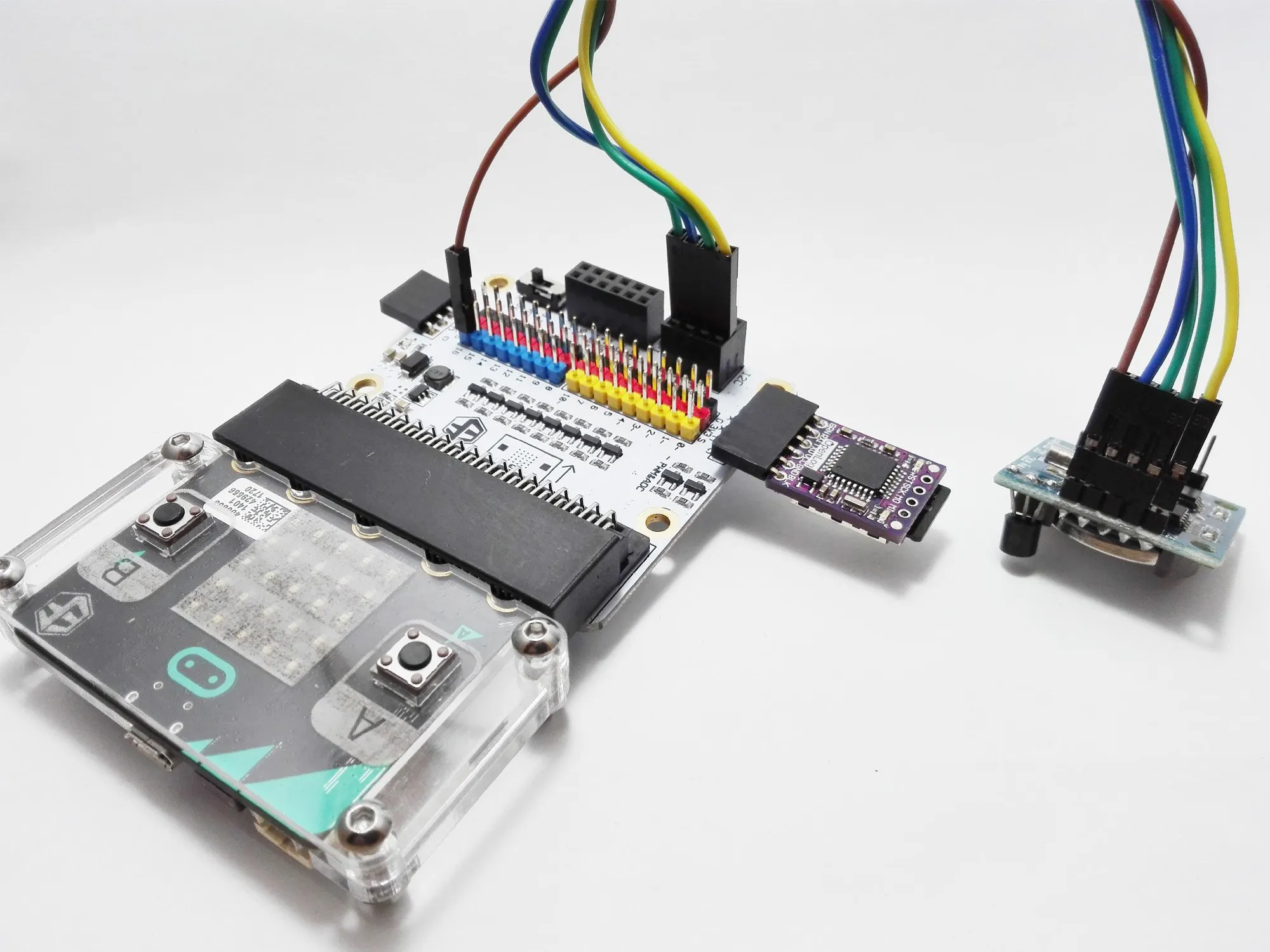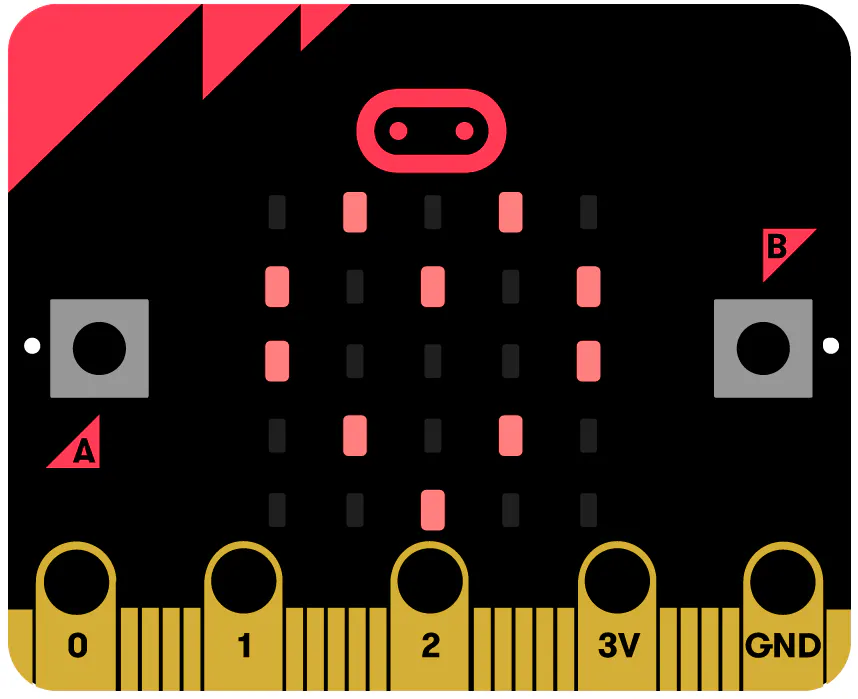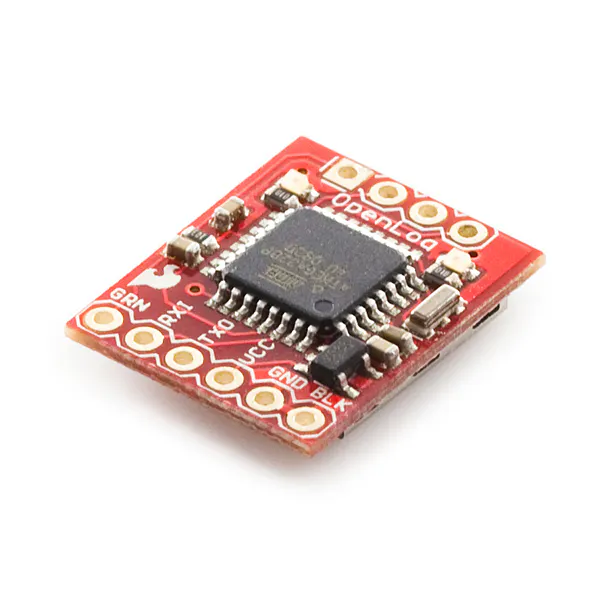# Temperature Data Logger

Measures and records temperature with BBC Micro:bit.

BeginnerFull instructions provided1 hour3,238## Things used in this project

### Hardware componentsBBC micro:bit board
×1
 ElecFreaks Micro:bit Breakout Board
×1
 ELECFREAKS Battery Holder With ON/OFF Switch and Cover for Micro:bit Board
×1SparkFun OpenLog
×1
 SparkFun microSD Card
×1
 ELECFREAKS Real-time Clock
×1
 ELECFREAKS DS18B20 Digital Temperature
×1

## Code

### Temperature Data Logger

Arduino
```#include <OneWire.h>
#include <Wire.h>    // I2C
#include "RTClib.h"  // RTC
#include <SoftwareSerial.h>
SoftwareSerial OpenLog(0, 8); 		// Serial DataLogger
RTC_DS1307 RTC;      				// RTC
#define TimeMin 5					// 5 Minute
#define DelayTime 60000*TimeMin
//OneWire DS18S20, DS18B20, DS1822 Temperature Example
OneWire ds(16);
void setup(void)
{
OpenLog.begin(9600);
Wire.begin();
RTC.begin();
Serial.begin(9600);
}
void loop(void)
{
byte i;
byte present = 0;
byte type_s;
byte data;
float celsius, fahrenheit;
{
ds.reset_search();
delay(250);
return;
}
{
Serial.println("CRC is not valid!");
return;
}
Serial.println();
// the first ROM byte indicates which chip
{
case 0x10:
type_s = 1;
break;
case 0x28:
type_s = 0;
break;
case 0x22:
type_s = 0;
break;
default:
Serial.println("Device is not a DS18x20 family device.");
return;
}
ds.reset();
ds.write(0x44, 1);        // start conversion, with parasite power on at the end
delay(1000);
present = ds.reset();
for ( i = 0; i < 9; i++)
{
}
// Convert the data to actual temperature
int16_t raw = (data << 8) | data;
if (type_s) {
raw = raw << 3; // 9 bit resolution default
if (data == 0x10)
{
raw = (raw & 0xFFF0) + 12 - data;
}
}
else
{
byte cfg = (data & 0x60);
if (cfg == 0x00) raw = raw & ~7;  // 9 bit resolution, 93.75 ms
else if (cfg == 0x20) raw = raw & ~3; // 10 bit res, 187.5 ms
else if (cfg == 0x40) raw = raw & ~1; // 11 bit res, 375 ms
}
DateTime now = RTC.now();   // time
show_time_and_date(now);  // time
celsius = (float)raw / 16.0;
fahrenheit = celsius * 1.8 + 32.0;
OpenLog.print(celsius);
OpenLog.println();
delay(DelayTime);
}
void show_time_and_date(DateTime datetime){
// Year/Month/Day
OpenLog.print(datetime.year(),DEC);
OpenLog.print("/");
if(datetime.month() < 10)
{
OpenLog.print(0);
}
OpenLog.print(datetime.month(),DEC);
OpenLog.print("/");
if(datetime.day() < 10)
{
OpenLog.print(0);
}
OpenLog.print(datetime.day(),DEC);
OpenLog.print(",");
// Hour:Minute:Second
if(datetime.hour() < 10)
{
OpenLog.print(0);
}
OpenLog.print(datetime.hour(),DEC);
OpenLog.print(":");
if(datetime.minute() < 10)
{
OpenLog.print(0);
}
OpenLog.print(datetime.minute(),DEC);
OpenLog.print(":");
if(datetime.second() < 10)
{
OpenLog.print(0);
}
OpenLog.print(datetime.second(),DEC);
OpenLog.print(",");
}
```

### Temperature Data Logger.xlsx

Plain text
`No preview (download only).`

## Credits

### Ramin Sangesari

3 projects • 306 followers
Programmer and IoT Developer